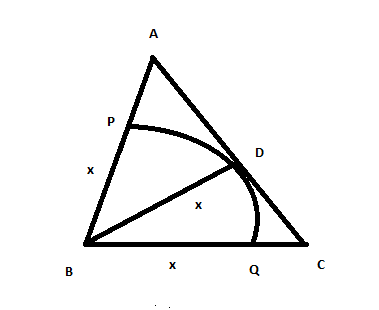Question 83

# In the triangle ABC, AB = 6, BC = 8 and AC = 10. A perpendicular dropped from B, meets the side AC at D. A circle of radius BD (with center B) is drawn. If the circle cuts AB and BC at P and Q respectively, the AP:QC is equal to

SolutionLet BD = x .Semi-perimeter of triangle ABC = 12. Now by herons formula area of ABC is 24. Also Area = 0.5*x*10 . We get x = 24/5 .  AP = 6/5  and CQ = 16/5 . Hence the required ratio is 3:8.